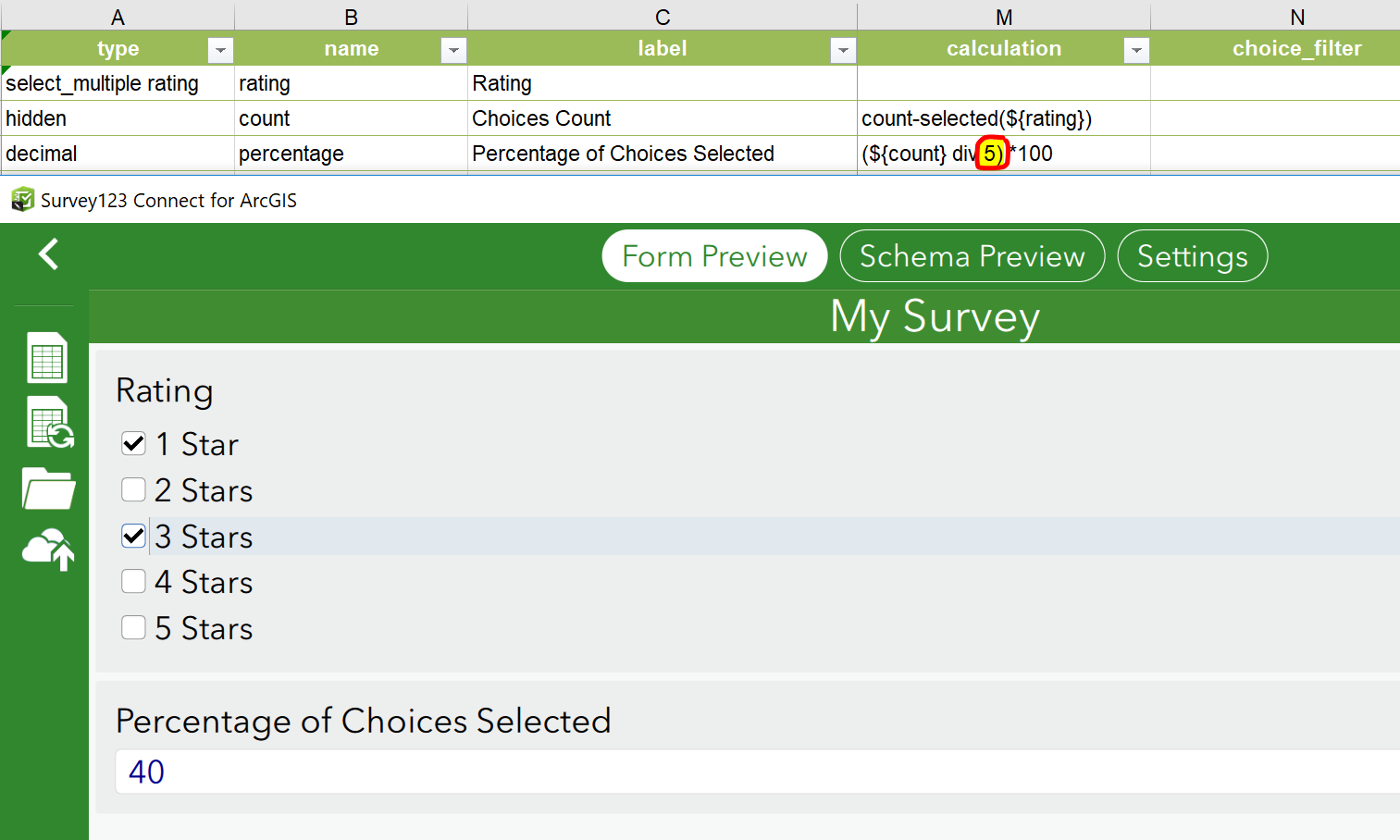Calculate a percentage based on selection from drop-down list

268
1
04-07-2019 05:51 AMNew Contributor II

Hi Everyone,

Is there a way to calculate a percentage based on the selection of choices in a drop-down list in Survey123. I use an XLSForm and would like to know if it is possible to calculate a percentage if the user checks multiple choices from the list of drop downs.

Any help in this regard would be greatly appreciated.byEsri Contributor

Hi Maneesh,

I'm not sure I understand your question. Are you looking to calculate the percentage of choices that are selected from a select_multiple question? If so, you can use the count-selected() function to count the number of choices from your list that have been selected. This can then just be divided by the number of possible choices in your list (which is 5 in the following example) and converted to percentage. The count question can be hidden if you like, for example:Hope this helps,

Brett